## teaching and educationJOURNAL OFAPPLIEDCRYSTALLOGRAPHY
ISSN: 1600-5767
Volume 51| Part 4| August 2018| Pages 1221-1225

## The rise and fall of Weber indices

aUniversité de Lorraine, CNRS, CRM2, Vandoeuvre-lès-Nancy F-54000, France
*Correspondence e-mail: massimo.nespolo@univ-lorraine.fr

(Received 6 March 2018; accepted 8 May 2018; online 12 June 2018)

Weber indices were introduced to provide a unique expression of a lattice direction with respect to the four-axis setting used for hexagonal and rhombohedral crystals. They are in general fractional indices, even in the case of a primitive hexagonal unit cell, but they are often carelessly reduced to integer values. This corresponds, on the one hand, to taking as direction indices the nodes of a lattice further from the origin and, on the other hand, to adopting a hybrid indexing between direct and reciprocal space. A critical analysis of the drawbacks of Weber indices is presented, which justifies the reluctance of crystallographers to adopt them, despite a more widespread use in fields like electron microscopy and metal science.

### 1. Introduction

As in the case of lattice planes, a four-index notation has been introduced for lattice directions (Weber, 1922), which is known as Weber indices' or Weber symbols'. Several critical analyses of this notation are available in the older literature (Hey, 1930; Wolfe, 1944; Donnay, 1947; Terpstra, 1927, 1952; Otte & Crocker, 1965). The use of Weber indices is relatively common in electron diffraction and metal science but much less in crystallography: they are not even mentioned in International Tables for Crystallography. The reason for this lack of success is that they are not […] suited for crystallographic calculation' (Bloss, 1971, p. 64). Nevertheless, they are mentioned in some textbooks, like those by Mittemeijer (2010), De Graef & McHenry (2012) – where they are incorrectly called Miller–Bravais direction indices' – and Hammond (2015), and in our experience it is not rare that students or newcomers to the field get confused about the meaning and use of four-digit indices. Also, questions sometimes appear on research-oriented social networks about the use of four-digit rather than three-digit indices, but the fundamental difference between Bravais–Miller indices and Weber indices is seldom, if ever, pointed out as it should be. In this article we show that, whereas the use of Bravais–Miller indices is fully justified when adopting hexagonal axes, Weber indices essentially represent an unnecessary complication. Moreover, the careless but common practice of systematically transforming Weber indices to integer numbers even when they are fractional leads to inconsistent and incorrect results.

We remind the reader that the direction indices of the zone axis of a set of lattice planes are proportional to the cross product of the vectors perpendicular to any pair of lattice planes belonging to the zone,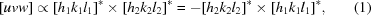and that the condition for a direction [uvw] to be contained in a lattice plane (hkl) is given by the Weiss law: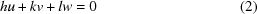(see, e.g. Bloss, 1971).

To save space, in the following discussion row matrices will be indicated as ( | (bra') and column matrices as | ) (ket').

### 2. Four-axis setting

Indexing of lattice planes with respect to hexagonal axes is usually performed using four basis vectors, of which three are in the plane perpendicular to the unique axis. Obviously, in a plane only two non-collinear vectors can be chosen as linearly independent; all the other vectors can be expressed as a linear combination of these two. Nevertheless, the additional, redundant, axis turns out to be quite useful to identify at first sight lattice planes that are symmetrically equivalent, as well as crystal faces that belong to the same crystal form.

A hexagonal lattice possesses seven symmetry directions. One is the unique axis (sixfold), usually taken as the  direction, and the other six are perpendicular to it, 30° apart from each other, defining two sets of equivalent directions: 〈100〉 (collective symbol for the three directions ,  and [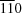]) and 〈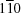〉 (collective symbol for the three directions [],  and [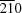]). These are called the primary, secondary and tertiary symmetry directions of the lattice. A rhombo­hedral lattice has four lattice directions; the tertiary symmetry directions of a hexagonal lattice are not compatible with rhombohedral centring.

Fig. 1(a) shows a stereographic projection of the symmetry elements of a point group of type 321, together with the hexagonal axes in the plane perpendicular to the unique axis.1 These axes are along the secondary symmetry directions and each of them is oriented along the bisector of the other two axes. To move from A1 to A2 we need a 120° rotation about the C axis, and if we continue to rotate in the same sense, we need a 240° rotation before we go back to A1. The third axis, A3, oriented along the third secondary direction, i.e. [], leads to basis vectors along all the secondary directions so that we find an axis every 120°. A3 is linearly dependent on A1 and A2, obeying the condition A1 + A2 + A3 = 0. We do not really need it, yet it is quite a useful companion.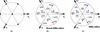Figure 1 (a) A stereographic projection of the symmetry elements of point group type 321. The three hexagonal axes A1, A2 and A3 in the plane perpendicular to the unique axis are shown. (b) A stereographic projection of the two forms {10.2} and {11.1} in the point group 321, with the corresponding Bravais–Miller indices of the faces. The permutation of indices h, k and i emphasizes the equivalence of these faces in the group. (c) The same as in panel (b) but with Miller indices only. The equivalence of the faces is less evident.

Hexagonal axes are used for both hexagonal and rhombo­hedral crystals. In the former case, (Bravais–)Miller indices and direction indices are relatively prime integer numbers. For rhombohedral crystals, instead, the hexagonal cell being rhombohedrally centred, (Bravais–)Miller indices are still integer numbers but they are not necessarily relatively prime; they obey the same restrictions as the reflection conditions. On the other hand, direction indices take fractional values when the first lattice node along the direction is not on a corner of the unit cell. For details, see Nespolo (2015, 2017).

### 3. Bravais–Miller indices

By adopting a four-axis setting we can assign four-digit indices to lattice planes. These four indices are labelled h, k, i and l and are called Bravais–Miller indices. The index i, which is obtained as a linear combination of h and k, i.e. i = −hk exactly like A3 = −A1A2 (the transformation of Miller indices is covariant with respect to a change of the basis), is sometimes replaced by a dot, so that Bravais–Miller indices are sometimes written as (hk.l). This makes it clear whether the writer is using hexagonal or rhombohedral axes; in the latter case, no dot occurs in the symbol of a lattice plane or crystal face.

The advantage of Bravais–Miller indices with respect to Miller indices is self-evident when indexing the faces belonging to a crystal form. Figs. 1(b) and 1(c) give two simple examples, for the forms {10.2} and {11.1} in the same group 321 shown in Fig. 1(a), where both forms have multiplicity six. The symmetry of the permutation of indices in the case of Bravais–Miller indices directly reflects the symmetry of the planes or faces, which is instead less evident in the case of Miller indices.

### 4. Weber indices

The adoption of a third axis in the plane perpendicular to the unique axis makes the direction indices dependent on the two axes that are chosen as independent. Fig. 2shows a lattice direction whose indices with respect to the A1, A2, C basis are . If we want to express this direction with respect to a basis with four vectors A1, A2, A3 and C, we simply need to add a zero in the third position. Indeed, the first lattice node in this direction is reached from the origin by taking a path of two lattice nodes along A1, three lattice nodes along A2 and no further movement along A3: the direction indices become . However, we can also take an alternative path of one lattice node along −A1 and three lattice nodes along −A3, so that the direction indices become [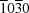]. A further alternative path consists of moving one lattice node along A2 and two lattice nodes along −A3, so that the direction indices become [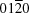]. The indexing of a lattice direction is therefore no longer unique when a basis with four vectors is adopted, contrary to the case of lattice planes, for which Bravais–Miller indexing is unique. The three direction indices make reference to a pair of axes Ai, Aj (ij), the third index being always zero, and all obey the Weiss law. Indeed, the Bravais–Miller indices of the lattice plane in the  zone that contains this direction are obtained starting from any of the three results above, by simply dropping the position of the zero index: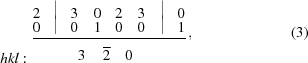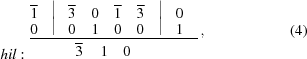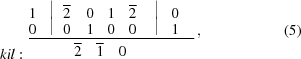so that the Bravais–Miller indices are (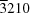) or (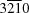). The Weiss law is verified for the three indexing: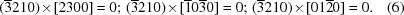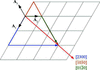Figure 2 The lattice direction  takes different indexing depending on the path chosen to reach the first lattice node on the direction from the origin. Three different paths are shown, each with no step along one of the three axes in the (0001) plane.

Although straightforward, this notation has the disadvantage of being non-unique. We can obtain further indexing for the same direction by adding the same number to the three indices corresponding to A1, A2 and A3. Let us start from [uv0w] and add a null path of j nodes; the corresponding four-index symbol is [u+j, v+j, j, w]. The additional null path does not influence the validity of the Weiss law, because its contribution is simply j(h + k + i) = 0. We can choose j so that the sum of the three indices is zero: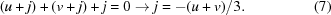The result is [u − (u + v)/3, v − (u + v)/3, −(u + v)/3, w] = [(2uv)/3, (2vu)/3, −(u + v)/3, w]. By putting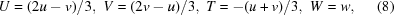we get a unique four-symbol indexing known as the Weber indices [UVTW] (Weber, 1922), which are in general no longer integer numbers even for primitive unit cells. Fig. 3shows again the example of the direction , re-indexed as , [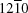] or [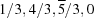]: these last are Weber indices. Unfortunately, the denominator 3 is usually dropped and the direction is then indexed as [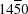], which corresponds to taking the third lattice node on the direction, contrary to the definition of direction indices. In other words, using [] as Weber indices is equivalent to using  as direction indices.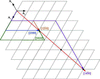Figure 3 Three different indexings of the lattice direction  obtained by adding the same integer number (0, 1 and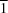) to the first three indices of . Weber indices were introduced to provide a unique path among the infinitely many possibilities. However, the careless practice of systematically transforming them to integer values leads to inconsistencies, as demonstrated by the indexing [] which, although satisfying the condition T = −U − V, corresponds to taking the coordinates of the third lattice along the direction, after the origin, as direction indices. This can be verified by converting [] back to [uvw], which gives  instead of .

The opposite relations are easily obtained as follows: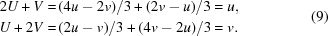The relations above are well known in the literature. There is, however, a connection that, to the best of our knowledge, seems to have been overlooked: as we are going to show, Weber indices, when carelessly transformed to integers, are actually a hybrid indexing between direct and reciprocal space.

In a general case, a direction in direct space [uvw] is not rational in reciprocal space, and vice versa a direction [hkl]* is not rational in direct space. We can nevertheless express these directions in dual space; if the result is an irrational direction, then the indices are not rational. This is obtained by imposing that the two expressions in dual space coincide: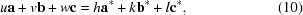which in row matrix/column matrix notation becomes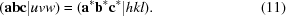If we now multiply both members by |abc) we obtain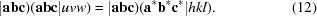|abc)(abc| is simply the metric tensor in direct space, G, whereas |abc)(a*b*c*| is 3I, where I is the identity matrix. To show that this is the case, we have to write out explicitly the expression of the matrices of the basis vectors. The matrices (abc| and (a*b*c*| give the components of the basis vectors with respect to a reference, which is normally chosen as a Cartesian basis e1, e2, e3,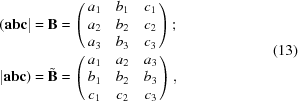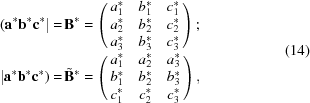where aj, bj and cj are the jth (j = 1, 2, 3) components of a, b and c, respectively, on ej, and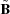and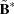are the transposed matrices of B and B* respectively. Accordingly, |abc)(abc| =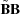= G and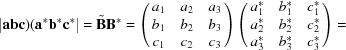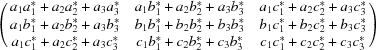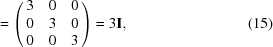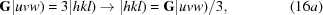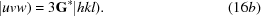Equation (16b) gives the [uvw] expression of [hkl]*, whereas equation (16a) gives the [hkl]* expression of [uvw]. In the case of hexagonal axes (a = b, α = β = 90°, γ = 120°), they simplify as follows: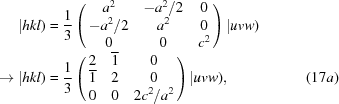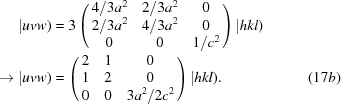From equation (17b) we obtain u = 2h + k, v = h + 2k, w = 3la2/2c2, and from equation (17a)we obtain h = (2uv)/3, k = (−u + 2v)/3, l = 2wc2/3a2. Comparison with equation (9)shows that U = h and V = k. However, wl (and thus Wl) unless l = 0 or c = a(3/2)1/2. In other words, there is a precise relation between the Weber indices of a direction in direct space and the indices of that direction in reciprocal space that can be summarized as follows:

(i) Weber indices are in general fractional, even when the unit cell is primitive; the indices in reciprocal space are instead always integer because the Miller indices of the corresponding lattice planes are always integer.

(ii) The fourth Weber index W is by definition the same as the third direction index w and different from the l index in direct space, unless l = 0 or in the case of the specialized metric c = a(3/2)1/2.

(iii) Weber indices must not, in general, be reduced to integer values, as is very often done, otherwise

(a) the coordinates of a lattice node further from the origin are taken as direction indices, contrary to the definition of direction indices; and

(b) the result corresponds to using a hybrid indexing, partly in reciprocal space (h and k) and partly in direct space [W = w ≠ l, unless l = 0 or c = a(3/2)1/2].

Let us consider the example of the  direction analysed above. From equation (8)we get [UVTW] = [] and from equation (17b) we get [hkl]* = * after multiplying by 3. If the Weber indices are made integer as well, then the result is precisely the expression of the direction  in reciprocal space once the index T is dropped, as shown in Fig. 4. If we now consider the direction  and repeat the same calculations, we get [UVTW] = [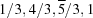], whereas [hkl]* = [1, 4, (2c2/a2)]* which is in general irrational, unless c = a(n/2)1/2, with n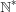. If the Weber indices are made integer as well, then the result [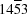] can be interpreted either as [uvw] = , which is not the correct direction index, or as *, which is not the same as [1, 4, (2c2/a2)]* unless c = a(3/2)1/2. In both cases, the result is incorrect.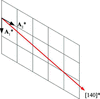Figure 4 Weber indices transformed to integer numbers correspond to expressing the lattice direction in a hybrid direct–reciprocal basis A1*, A2*, C. For the case l = 0, as in the example shown here, because W = w = 0 – as well as for the case of metric specialization c = a(3/2)1/2 (not shown) – this corresponds to adopting a consistent indexing in reciprocal space. However, for the general case the result is inconsistent and incorrect.

### 5. Conclusions

Weber indices [UVTW] were introduced long ago to provide a unique expression of a lattice direction with respect to the four-axis setting used for hexagonal and rhombohedral crystals. They are in general fractional indices, even in the case of a primitive hexagonal unit cell, contrary to the usual indices [uvw] which are always integer for primitive cells. If Weber indices are used, no attempt should ever be made to modify the indices arbitrarily; the careless but common practice of reducing fractional Weber indices to integer values is incorrect for two reasons. Firstly, it corresponds to taking as direction indices the nodes of a lattice further from the origin, as can be immediately verified by converting them back to direction indices [uvw]. Secondly, the result corresponds to adopting a hybrid indexing between reciprocal (U = h, V = k) and direct (W = w) space and to using a hybrid axial setting with basis vectors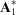,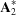and C, unless c = a(3/2)1/2. For the special case of lattice directions perpendicular to a lattice plane, which are rational in both direct and reciprocal space, i.e. for the direction [uvw] =  or [uv0], the Weber indices become  = * and [UVT0] = [hk0]*, i.e. they correspond to indexing in reciprocal space. Now, in electron microscopy the directions along which the electron beam is aligned are low-index zone axes, which for hexagonal or rhombohedral crystals in hexagonal axes are precisely  or [UVT0]. This means that, in practice, the zone axis is expressed through the reciprocal lattice indices [hk0]* instead of the direct lattice indices [uv0]. For general directions, Weber indices represent instead an unnecessary complication that `should be discontinued' (Bloss, 1971).

### Footnotes

1It is quite common to use upper-case letters to label hexagonal axes, to distinguish them clearly from rhombohedral axes, labelled by lower-case letters.

### Acknowledgements

Copies of the relevant pages from the rare textbook in Dutch by Terpstra (1927) were kindly provided by Dr Bernd Souvignier (Radboud University Nijmegen, The Netherlands). The author thanks Professor Peter Zeiner (Bielefeld University, Germany) for useful discussion that led to a more rigorous derivation of equations (16a) and (16b). Critical remarks by three anonymous reviewers are gratefully acknowledged.

### ReferencesJOURNAL OFAPPLIEDCRYSTALLOGRAPHY